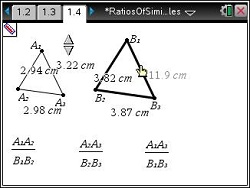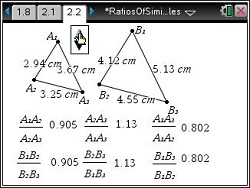# Activities

••• ##### Subject Area

• Math: Algebra I: Expressions

• ##### Author9-12

20 Minutes

• ##### Device
•TI-Nspire™ CX/CX II
•TI-Nspire™ CX CAS/CX II CAS
• TI-Nspire™
• TI-Nspire™ CAS
• ##### Software

TI-Nspire™
TI-Nspire™ CAS

3.2

## Ratios of Similar Triangles

#### Activity Overview

In this activity, students will explore two ways of comparing side lengths of similar triangles. They will calculate ratios and change the triangles to see how the ratio changes. Then they will write proportions using the ratios.

#### Key Steps

•On page 1.4, students will find two similar triangles (A and B). They need to find the lengths of each side of both triangles using the Length tool (MENU > Measurement > Length). Then students can use the Calculate tool (MENU > Actions > Calculate) to find the ratios of corresponding sides.

•On page 2.2, students will find the same two similar triangles.  They need to find the lengths of the sides of both triangles.  Students can use the Calculate tool to find the ratios of two segments in a triangle.